ISEE Middle Level Math : How to find percentage

Example Questions

Example Question #91 : Percentage

What is the equivalent of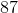%?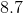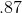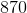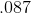Explanation:

To solve, divide: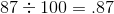Answer:Example Question #92 : Percentage

What is the equivalent of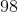%?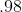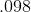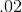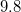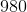Explanation:

To solve, divide: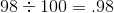Answer:Example Question #93 : Percentage

What is the equivalent of%?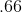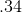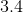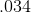Explanation:

Divide: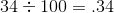Answer:Example Question #94 : Percentage

What is the equivalent of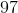%?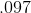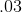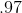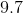Explanation:

Divide: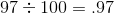Answer:Example Question #95 : Percentage

What is the equivalent of%?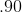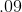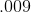Explanation:

Divide: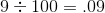Answer:Example Question #96 : Percentage

What is the equivalent of%?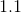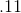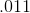Explanation:

Divide: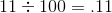Answer:Example Question #97 : Percentage

What is the equivalent of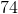%?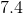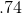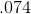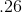Explanation:

Divide: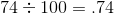Answer:Example Question #98 : Percentage

What is the equivalent of%?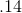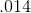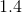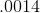Explanation:

Divide: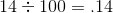Answer:Example Question #99 : Percentage

What is the equivalent of%?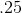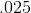Explanation:

Divide: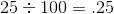Answer:Example Question #100 : Percentage

What is the equivalent of%?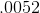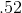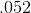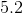Divide: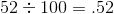Answer: